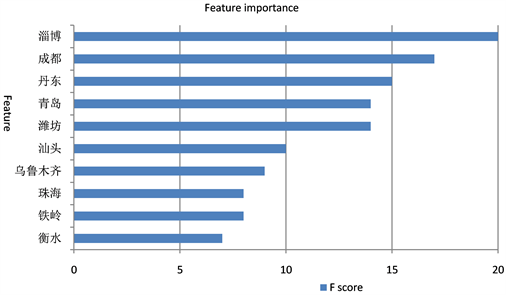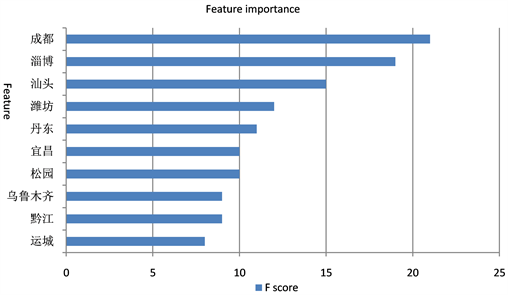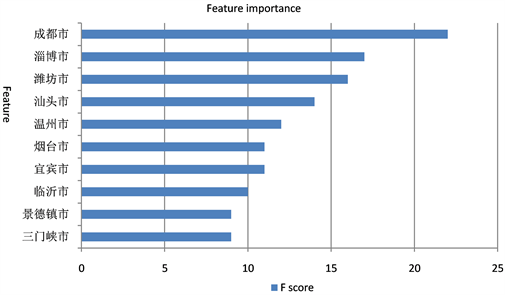# 基于模型融合的互联网信贷信用风险预测研究Research on Internet Credit Risk Prediction Based on Model Fusion

DOI: 10.12677/SA.2019.85093, PDF, HTML, XML, 下载: 404  浏览: 1,232  科研立项经费支持

Abstract: The prediction of the credit risk of Internet credit is a key factor for the sustainable development of Internet finance. It can accurately estimate the credit risk of borrowers before lending, effectively reducing the possible risk loss of enterprises. With the development of machine learning, the algorithm model of machine learning has been applied more and more in the credit risk of Internet credit. In order to explore the effect of integrating tree model and linear model in the prediction of credit risk of Internet credit, this paper adopts Stacking model fusion method to design the credit risk prediction model, in which the first layer model is random forest, XGBoost and LightGBM and the second layer model is logistic regression, and conducts experiments on the real data of Clap to Borrow. Compared with the performance of the single model on AUC, accuracy and time consuming, the results show that the fused model, although takes longer time, but performs better in terms of AUC and accuracy, which provides a new idea for the construction of financial credit risk prediction model.

1. 引言

2. 模型介绍

2.1. 逻辑回归模型

$P\left(Y=1|x\right)=\pi \left(x\right),\text{\hspace{0.17em}}\text{\hspace{0.17em}}P\left(Y=0|x\right)=1-\pi \left(x\right)$ (1)

${\prod }_{i=1}^{N}{\left[\pi \left({x}_{i}\right)\right]}^{{y}_{i}}{\left[1-\pi \left({x}_{i}\right)\right]}^{\left(1-{y}_{i}\right)}$ (2)

$\begin{array}{c}L\left(w\right)=\underset{i=1}{\overset{N}{\sum }}\left[{y}_{i}\mathrm{log}\pi \left({x}_{i}\right)+\left(1-{y}_{i}\right)\mathrm{log}\left(1-\pi \left({x}_{i}\right)\right)\right]\\ =\underset{i=1}{\overset{N}{\sum }}\left[{y}_{i}\mathrm{log}\frac{\pi \left({x}_{i}\right)}{1-\pi \left({x}_{i}\right)}+\mathrm{log}\left(1-\pi \left({x}_{i}\right)\right)\right]\\ =\underset{i=1}{\overset{N}{\sum }}\left[{y}_{i}\left(w\ast {x}_{i}\right)-\mathrm{log}\left(1+\mathrm{exp}\left(w\ast {x}_{i}\right)\right)\right]\end{array}$ (3)

$P\left(Y=1|x\right)=\frac{{\text{e}}^{\stackrel{¯}{w}x}}{1+\left({\text{e}}^{\stackrel{¯}{w}x}\right)}$ (4)

$P\left(Y=0|x\right)=\frac{1}{1+\left({\text{e}}^{\stackrel{¯}{w}x}\right)}$ (5)

2.2. 随机森林模型

2.3. XGBoost模型

XGBoost模型是Chen等人在2016年提出的一种Boosting模型 ，在传统GBDT算法的基础上，对损失函数进行二阶泰勒展开，并且加入了正则化项，平衡模型的复杂度和的目标函数的下降速度，能够有效解决过拟合问题。Boosting的思想是将多个弱学习分类器集合起来形成一个强学习分类器，而XGBoost所用到的树模型是CART树。XGBoost通过不断的添加树来分裂特征，每添加一棵树就是在学习一个新函数来拟合上个函数的残差。在对数据进行预测时，就是这个数据的每个特征都会落到一个叶子结点上，最后的输出值就是这些树的分数的和。XGBoost的算法模型如公式(6)所示，xi为第i个样本的特征向量，fk是一个回归树，F是回归树的集合。

$\stackrel{¯}{{y}_{i}}={\sum }_{k=1}^{K}{f}_{k}\left({x}_{i}\right)\text{\hspace{0.17em}},\text{\hspace{0.17em}}\text{\hspace{0.17em}}{f}_{k}\in F$ (6)

$Ob{j}^{\left(t\right)}={\sum }_{i=1}^{n}l\left({y}_{i},{\stackrel{¯}{y}}_{i}^{\left(t\right)}\right)+{\sum }_{i=1}^{t}\theta \left({f}_{i}\right)$ (7)

XGBoost一次添加一棵树，整个优化流程如下：

${\stackrel{¯}{y}}_{i}^{\left(0\right)}=0$

${\stackrel{¯}{y}}_{i}^{\left(1\right)}={f}_{1}\left({x}_{i}\right)={\stackrel{¯}{y}}_{i}^{\left(0\right)}+{f}_{1}\left(xi\right)$

${\stackrel{¯}{y}}_{i}^{\left(2\right)}={f}_{1}\left({x}_{i}\right)+{f}_{2}\left({x}_{i}\right)={\stackrel{¯}{y}}_{i}^{\left(1\right)}+{f}_{2}\left(xi\right)$

$\cdots$

${\stackrel{¯}{y}}_{i}^{\left(t\right)}={\sum }_{k=1}^{t}{f}_{k}\left({x}_{i}\right)={\stackrel{¯}{y}}_{i}^{\left(t-1\right)}+{f}_{t}\left({x}_{i}\right)$ (8)

$Ob{j}^{\left(t\right)}={\sum }_{i=1}^{n}l\left({y}_{i},{\stackrel{¯}{y}}_{i}^{\left(t-1\right)}+{f}_{t}\left({x}_{i}\right)\right)+\theta \left({f}_{t}\right)+C$ (9)

XGBoost的思想是在 ${f}_{t}=0$ 的二阶泰勒展开来求得近似值，引入正则项后，得：

$Ob{j}^{\left(t\right)}={\sum }_{i=1}^{n}\left[{g}_{i}{f}_{t}\left({x}_{i}\right)+\frac{1}{2}{h}_{i}{f}_{t}^{2}\left({X}_{i}\right)\right]+\theta \left({f}_{t}\right)$ (10)

2.4. LightGBM模型

LightGBM是由微软亚洲研究院在2017年提出的一种GBDT框架 ，相较于传统的GBDT算法，LightGBM的优化主要包括三部分：基于Histogram的决策树算法、带有深度限制的Leaf-wise的叶子生长策略、直方图做差加速，既提升了算法的效率，又能防止过拟合。

Histogram算法的基本思想是先把连续的浮点特征值离散化为k个整数，同时构造一个宽度为k的直方图。在遍历数据的时候，根据离散化后的值作为索引在直方图中累计统计量，当遍历一次数据后，直方图累积了需要的统计量，然后根据脂肪乳的离散值，遍历寻找最优的分割点。

3. 实验过程

3.1. 实验数据和描述Table 1. User behavior information tableTable 2. User login data tableTable 3. User information update table

3.2. 数据预处理

1) 数据清洗Figure 1. Number of missing value attributes for each sample

2) 特征工程Figure 2. City importance rankingFigure 3. City importance rankingFigure 4. City importance rankingFigure 5. City importance ranking

3) 样本均衡

4. 模型训练

4.1. 模型调参与融合

4.2. 模型评价标准

5. 实验结果

5.1. 模型调参结果Table 6. Compare before and after adjusting parameters

XGBoost、随机森林、LightGBM三个算法模型的最优参数分别如表7表8表9所示：Table 7. The optimal parameter of XGBoostTable 8. The optimal parameter of random forestsTable 9. The optimal parameter of LightGBM

5.2. 不同算法模型结果对比分析Table 10. Performance comparison of different models

6. 总结

  于晓虹, 楼文高. 基于随机森林的P2P网贷信用风险评价、预警与实证研究[J]. 金融理论与实践, 2016(2): 53-58.  Redmond, U. and Cunningham, P. (2013) A Temporal Network Analysis Reveals the Unprofitability of Arbitrage in the Prosper Marketplace. Expert Systems with Applications, 40, 3715-3721. https://doi.org/10.1016/j.eswa.2012.12.077  Malekipirbazari, M. and Aksakalli, V. (2015) Risk Assessment in Social Lending via Random Forests. Expert Systems with Applications, 42, 4621-4631. https://doi.org/10.1016/j.eswa.2015.02.001  李昕, 戴一成. 基于BP神经网络的P2P网贷借款人信用风险评估研究[J]. 武汉金融, 2018(2): 33-37.  Ke, G.L., Meng, Q., Finley, T., Wang, T.F., Chen, W., Ma, W.D., Ye, Q.W. and Liu, T.-Y. (2017) LightGBM: A Highly Efficient Gradient Boosting Decision Tree. Advances in Neural Information Processing Systems, 30, 3149-3157.  李航. 统计学习方法[M]. 北京: 清华大学出版社, 2012: 78-79.  Verikas, A., Gelzinis, A. and Bacauskiene, M. (2011) Mining Data with Random Forests: A Survey and Results of New Tests. Pattern Recognition, 44, 330-349. https://doi.org/10.1016/j.patcog.2010.08.011  Chen, T. and Guestrin, C. (2016) XGBoost: A Scalable Tree Boosting System. Proceedings of the 22nd ACM SIGKDD International Conference on Knowledge Discovery and Data Mining, San Francisco, August 13-17, 2016, 785-794. https://doi.org/10.1145/2939672.2939785  Sun, Y., Wong, A.K.C. and Kamel, M.S. (2009) Classification of Imbalanced Data: A Review. International Journal of Pattern Recognition and Artificial Intelligence, 23, 687-719. https://doi.org/10.1142/S0218001409007326  Chawla, N.V., Bowyer, K.W., Hall, L.O., et al. (2002) SMOTE: Synthetic Minority Over-Sampling Technique. Journal of Artificial Intelligence Research, 16, 321-357. https://doi.org/10.1613/jair.953  Ling, C.X., Huang, J. and Zhang, H. (2003) AUC: A Better Measure than Accuracy in Comparing Learning Algorithms: Advances in Artificial Intelligence. 16th Conference of the Canadian Society for Computational Studies of Intelligence, AI 2003, Halifax, 11-13 June, 2003.